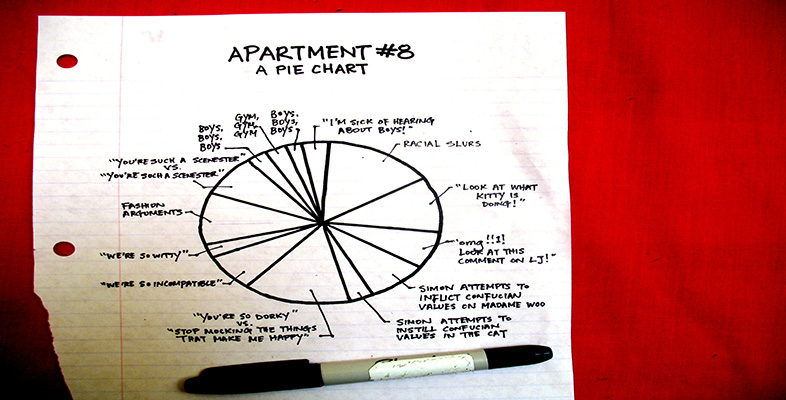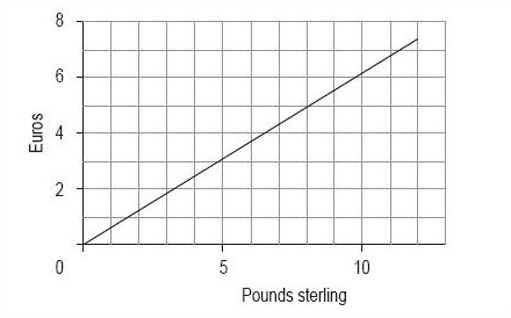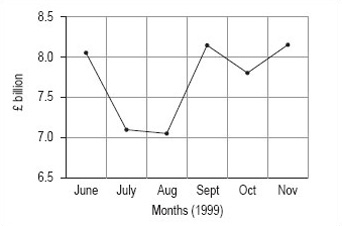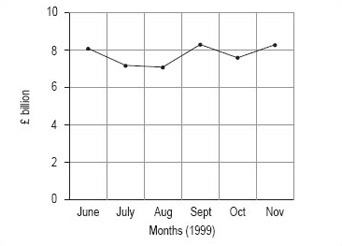More working with charts, graphs and tables

Start this free course now. Just create an account and sign in. Enrol and complete the course for a free statement of participation or digital badge if available.

Free course

# 4.2 Line graphs: Activities

## Activity 5

Line graphs are commonly used to show a direct relationship between data. For example, graphs for conversions between one amount and another, such as degrees Celsius and degrees Fahrenheit.

You should now follow our calculation of a line graph from the information in Table 1.

### Table 1 Conversion of pounds sterling to euros based on the exchange rate in February 2000

CurrencyEquivalent units
£12.5478.61012
euro (to 3 d.p)0.6231.5552.4884.3545.3496.2207.464
1. Collect the data.

The data is given in Table 1.

2. Decide on a clear title.

Conversion of pounds sterling to euros (February 2000).

3. Decide on the values that you wish to show on the horizontal axis and the vertical axis.

As this is a conversion from pounds to euros, the number of euros is dependent on the number of pounds. Therefore, euros are the dependent values and pounds are the independent values, so you should plot pounds on the horizontal axis and euros on the vertical axis. Remember to label the axes clearly.

4. Decide on the scale.

Given the data, it looks sensible to begin both axes from zero. The horizontal axis needs to go up to at least 12, so a sensible scale is 0 to 13 in steps of one. The vertical axis needs to go up to almost 8, so a likely scale is 0 to 8 in steps of one.

5. Plot the graph (Figure 1).Figure 1 Conversion of pounds sterling to euros (February 2000)

## Activity 6

When line graphs are drawn, the scale can often be misleading. Sometimes the graph seems to show something rather different from the data. This may be deliberate, to make a point, but sometimes it is accidental, perhaps because the author does not understand the best way to draw a graph.

To show you what we mean, we would like you to use the data in Table 2 to draw two line graphs – both for the total turnover from June to November 1999, but one with the vertical axis starting at £6.5 billion and the other with it starting at zero.

### Table 2 Total engineering turnover figures at current prices (£ billion)

1999Total turnover (£ billion)Home turnover (£ billion)Export turnover (£ billion)
June8.14.63.5
July7.24.23.0
August7.14.22.8
September8.34.83.5
October7.64.43.2
November8.34.93.5
Source: Office for National Statistics, Engineering Turnover and Orders, November 1999

### Discussion

1. Collect the data.

You have the data in the table, and you wish to show total turnover against time.

2. Decide on a clear title.

In this case, the heading of Table 2 seems to be suitable.

3. Decide on the values that you wish to show on the horizontal axis and the vertical axis.

The independent variable in this case is time, so you need to plot it on the horizontal axis. As the data in Table 2 is in months, use these for the graph. The dependent value is the total turnover, so you need to plot it on the vertical axis, in £ billion. Remember to label the axes.

4. Decide on the scale.

You should use months on the horizontal axis in both line graphs. In the first line graph, you should start the vertical axis at 6.5, just below the minimum value; in the second line graph, you should start the vertical axis at zero.

5. Plot the graph.

Using 6.5 as the starting point on the vertical axis, your graph should look like Figure 2.

Using zero as the starting point on the vertical axis, your graph should look like Figure 3.Figure 2: Total engineering turnover figures at current prices (£ billion)Figure 3: Total engineering turnover figures at current prices (£ billion)

## Activity 7

What difference can you see between the two graphs you drew in Activity 6?

### Discussion

The obvious difference is that change between the months seems to be much greater in the graph where the vertical axis starts at £6.5 billion. This is because the total scale on the vertical axis is much smaller than in the second graph, where the vertical axis starts at 0. If you wished to use the line graph to show that turnover is fluctuating strongly, you might prefer to use the first graph, whereas if you wished to suggest that the fluctuations were not overly strong, you might prefer the second graph.

Take a look around you at the line graphs (and other graphs and diagrams) that you see, and notice how many of them have a particular emphasis because of a misleading use of scale.

LDT_4